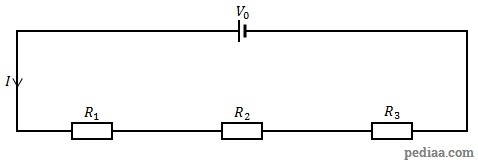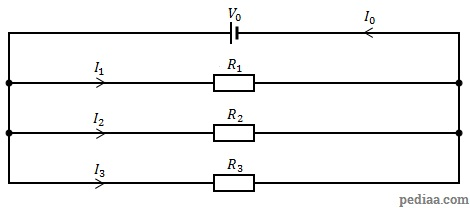# Difference Between Series and Parallel Circuits

## Main Difference – Series vs. Parallel Circuits

Components in a circuit can be connected in series or in parallel. The main difference between series and parallel circuits is that, in series circuits, all components are connected in series so that they all share the same current whereas, in parallel circuits, components are connected in parallel so that they all have the same potential difference between them.

## What are Series Circuits

In series circuits, there are no junctions between components. Then, according to the law of conservation of charge (Kirchoff’s first law), they have the same current flowing through them. If the circuit breaks at any point, current stops flowing through the circuit altogether.The following diagram shows a circuit, where three resistors are connected in series with the cell.A Series Circuit

## Resistors in Series

When resistors with resistances$R_1,R_2,R_3,\cdots ,R_N$ are connected in series, as shown above, the combined resistance$R_{tot}$ is given by:$R_{tot}=R_1+R_2+R_3+\cdots +R_N$

In series circuits, the power dissipated across a resistor is proportional to its resistance. Ammeters, which measure current, should have very little effect on the current. Therefore, they are made to have very small resistances and should e connected in series with the component.

## Inductors in Series

When inductors with inductances$L_1,L_2,L_3,\cdots ,L_N$ are connected in series, the combined inductance$L_{tot}$ is given by:$L_{tot}=L_1+L_2+L_3+\cdots +L_N$

## Capacitors in Series

When capacitors with capacitances$C_1,C_2,C_3,\cdots ,C_N$ are connected in series, the combined capacitance$C_{tot}$ is given by:$\frac{1}{C_{tot}}=\frac{1}{C_1}+\frac{1}{C_2}+\frac{1}{C_3}+\cdots +\frac{1}{C_N}$

## What are Parallel Circuits

In parallel circuits, a loop can be traced between two components without encountering other components along the loop. Then, according to the law of conservation of energy (Kirchoff’s second law) they have the same potential difference across them. If one branch of the circuit breaks, the other branches can still function. In the following diagram, the resistors are connected in parallel:A Parallel Circuit

## Resistors in Parallel

When resistors with resistances$R_1,R_2,R_3,\cdots ,R_N$ are connected in parallel, as shown above, the combined resistance$R_{tot}$ is given by:$\frac{1}{R_{tot}}=\frac{1}{R_1}+\frac{1}{R_2}+\frac{1}{R_3}+\cdots +\frac{1}{R_N}$

When resistors are connected in parallel, the power dissipated across a resistor is inversely proportional to its resistance. Voltmeters, which measure potential differences, are always connnected in parallel to the components.

## Inductors in Parallel

When inductors with inductances$L_1,L_2,L_3,\cdots ,L_N$ are connected in parallel, the combined inductance$L_{tot}$ is given by:$\frac{1}{L_{tot}}=\frac{1}{L_1}+\frac{1}{L_2}+\frac{1}{L_3}+\cdots +\frac{1}{L_N}$

## Capacitors in Parallel

When capacitors with capacitances$C_1,C_2,C_3,\cdots ,C_N$ are connected in parallel, the combined capacitance$C_{tot}$ is given by:$C_{tot}=C_1+C_2+C_3+\cdots +C_N$

## Difference Between Series and Parallel Circuits

### Current

In series circuits, the current flowing through the components is the same.

In parallel circuits, the current flowing through each branch depends on the impedance of components in that branch.

### Potential Difference

In series circuits, the magnitude of the potential difference across a component depends on the component’s impedance.

In parallel circuits, the magnitude of the potential difference across each component is the same.

### Resistance/Inductance

In series circuits, the resistances/inductances of individual resistors/inductors add up, so that the total resistance/inductance is always greater than the largest resistance/inductance of an individual resistor/inductor.

In parallel circuits, the total resistance/inductance of resistors/inductors is always smaller than the smallest resistance/inductance of an individual resistor/inductor.

### Capacitance

In series circuits, the total capacitance of capacitors is always smaller than the smallest capacitance of an individual capacitor.

In parallel circuits, the capacitance of individual capacitors add up, so that the total capacitance is always greater than the largest capacitance of an individual capacitor.